# Exponents for Experts - Set 1

### Explanation:

Though these problems look difficult, the approach is to use a simple solution of setting the exponents equal to each other.

### Example(s):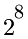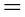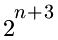Bases are equal, so.... 8 = n + 3 Solve this 1-step equation, so n = 5.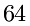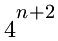1st: Make the bases equal.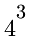Bases are equal, so 3 = n + 2. Answer: n = 1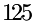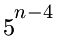1st: Make the bases equal.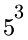Bases are equal, so 3 = n - 4. Answer: n = 7

### Directions:

Make the bases equal if necessary. Then set the exponents equal and solve the equation. Strive to understand and accomplish the problems with ease and then strive for increasing your speed. Good luck and enjoy the challenge!

...